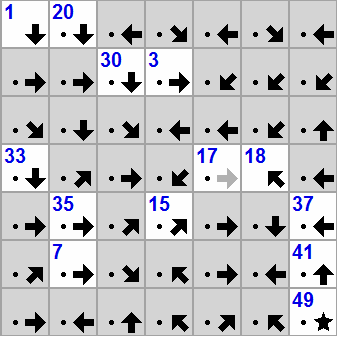# Which Arrow you should follow

Logic Level 4You have a grid of squares. The arrow on square $n$ points in the direction of square $n+1$, but you do not know the distance between them.

Find the integer of the square in the center of the grid

This problem is part of this Set

×